# Chapter 7 Chemical Reactions 1 All chemical reactions

• Slides: 56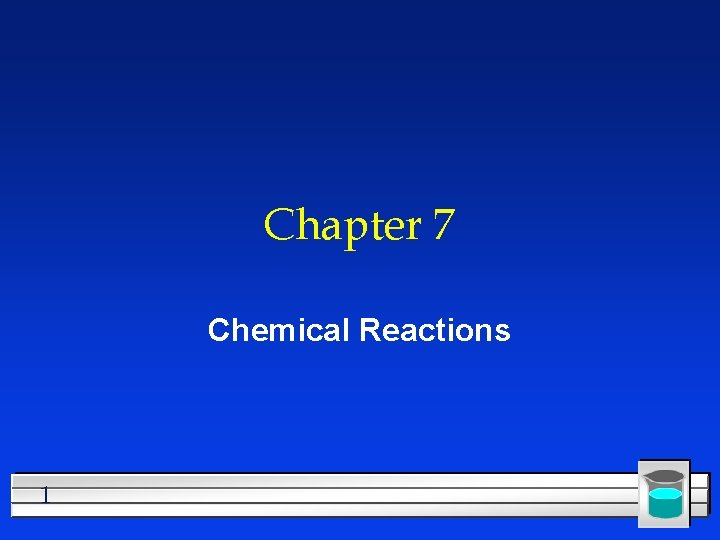Chapter 7 Chemical Reactions 1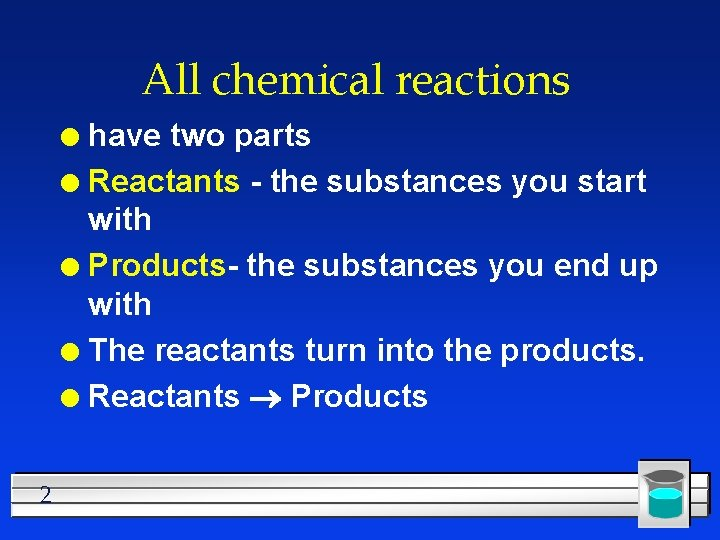All chemical reactions have two parts l Reactants - the substances you start with l Products- the substances you end up with l The reactants turn into the products. l Reactants Products l 2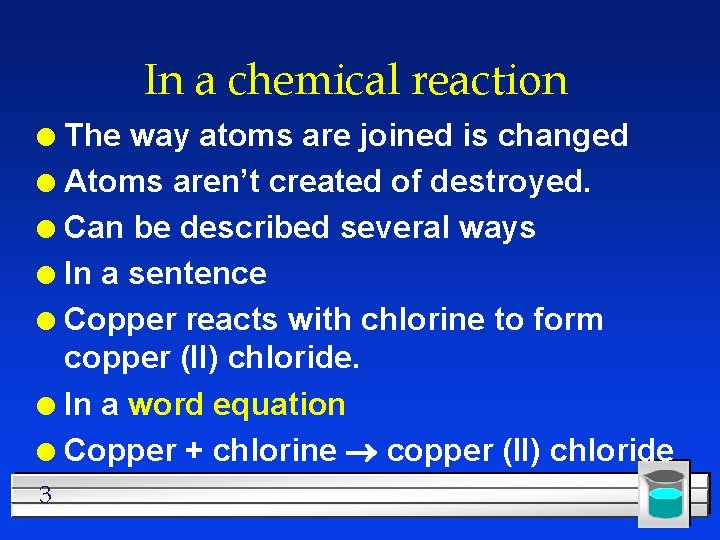In a chemical reaction The way atoms are joined is changed l Atoms aren’t created of destroyed. l Can be described several ways l In a sentence l Copper reacts with chlorine to form copper (II) chloride. l In a word equation l Copper + chlorine copper (II) chloride l 3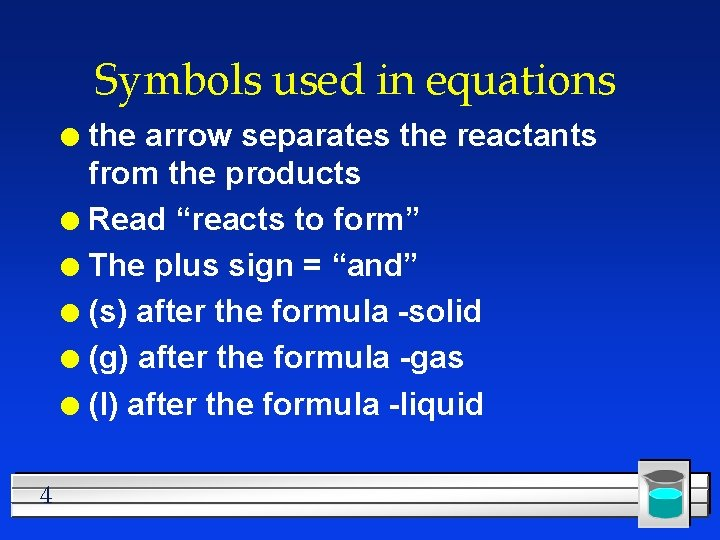Symbols used in equations the arrow separates the reactants from the products l Read “reacts to form” l The plus sign = “and” l (s) after the formula -solid l (g) after the formula -gas l (l) after the formula -liquid l 4Symbols used in equations (aq) after the formula - dissolved in water, an aqueous solution. l used after a product indicates a gas (same as (g)) l ¯ used after a product indicates a solid (same as (s)) l 5Symbols used in equations indicates a reversible reaction (More later) l shows that heat is supplied to the reaction l is used to indicate a catalyst used supplied, in this case, platinum. l 6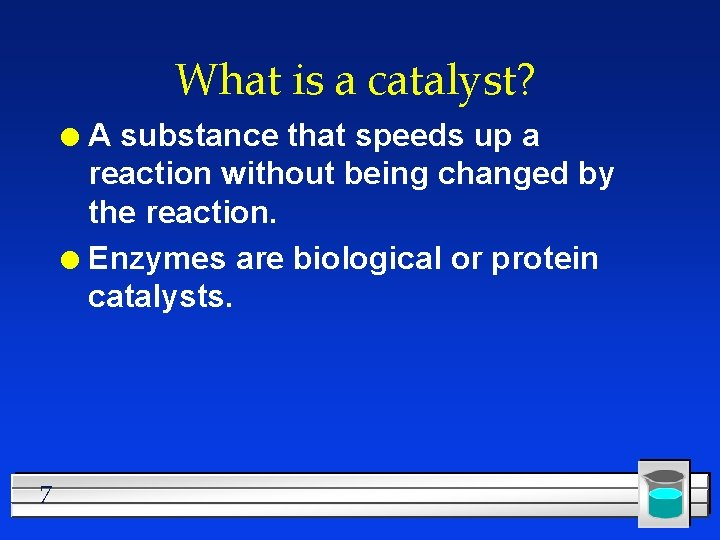What is a catalyst? A substance that speeds up a reaction without being changed by the reaction. l Enzymes are biological or protein catalysts. l 7Diatomic elements There are 8 elements that never want to be alone. l They form diatomic molecules. l H 2 , N 2 , O 2 , F 2 , Cl 2 , Br 2 , I 2 , and At 2 l The –ogens and the –ines l 1 + 7 pattern on the periodic table l 89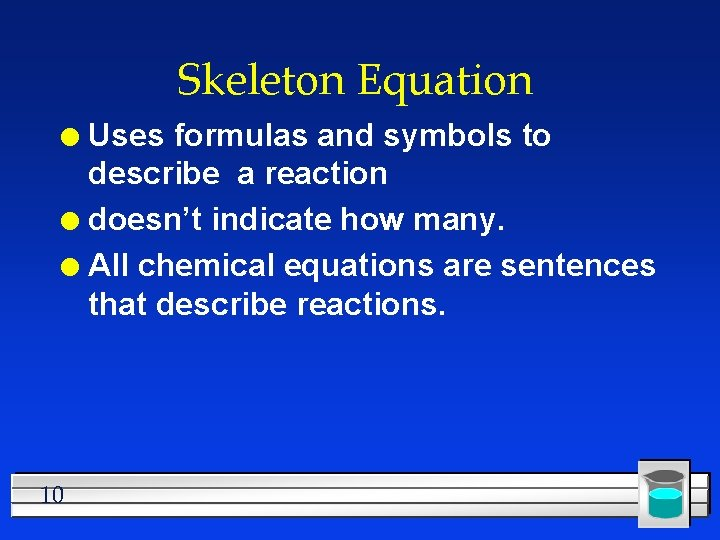Skeleton Equation Uses formulas and symbols to describe a reaction l doesn’t indicate how many. l All chemical equations are sentences that describe reactions. l 10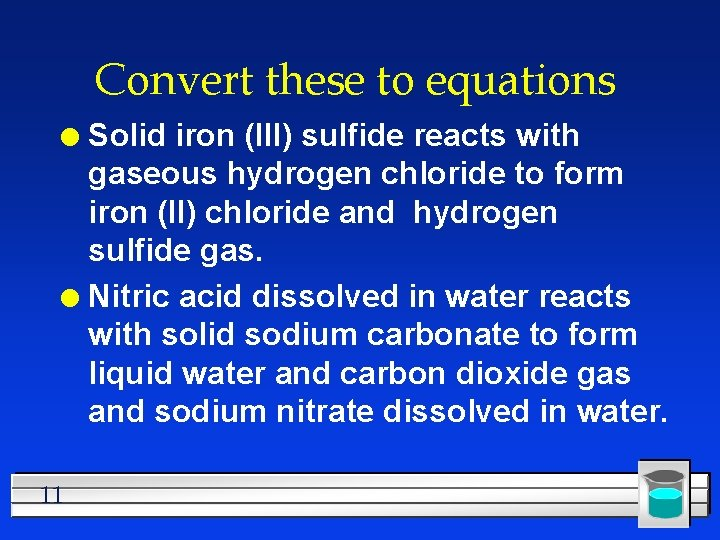Convert these to equations Solid iron (III) sulfide reacts with gaseous hydrogen chloride to form iron (II) chloride and hydrogen sulfide gas. l Nitric acid dissolved in water reacts with solid sodium carbonate to form liquid water and carbon dioxide gas and sodium nitrate dissolved in water. l 11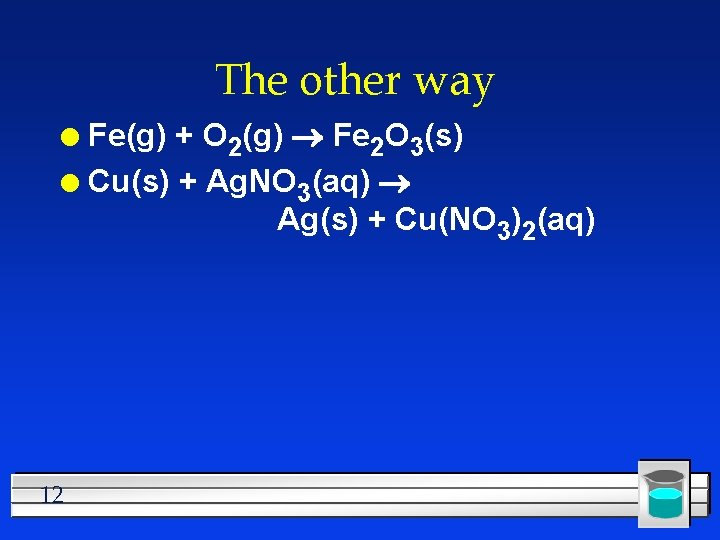The other way Fe(g) + O 2(g) Fe 2 O 3(s) l Cu(s) + Ag. NO 3(aq) Ag(s) + Cu(NO 3)2(aq) l 12Balancing Chemical Equations 13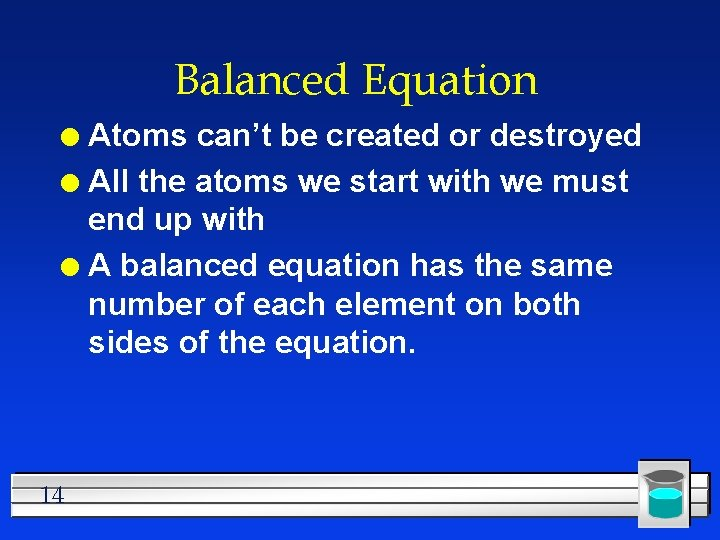Balanced Equation Atoms can’t be created or destroyed l All the atoms we start with we must end up with l A balanced equation has the same number of each element on both sides of the equation. l 14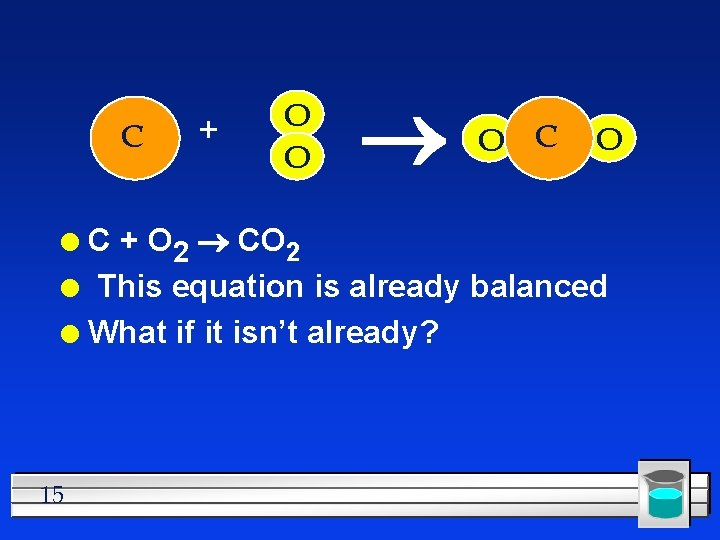C + O O O C + O 2 CO 2 l This equation is already balanced l What if it isn’t already? l 15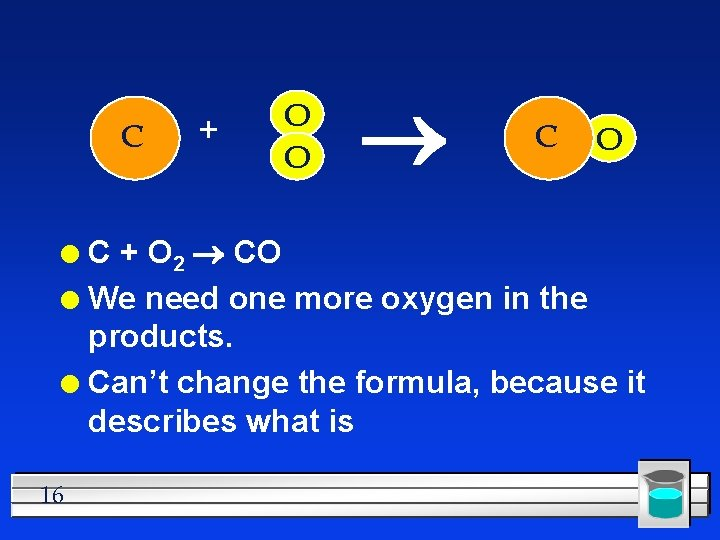C + O O C + O 2 CO l We need one more oxygen in the products. l Can’t change the formula, because it describes what is l 16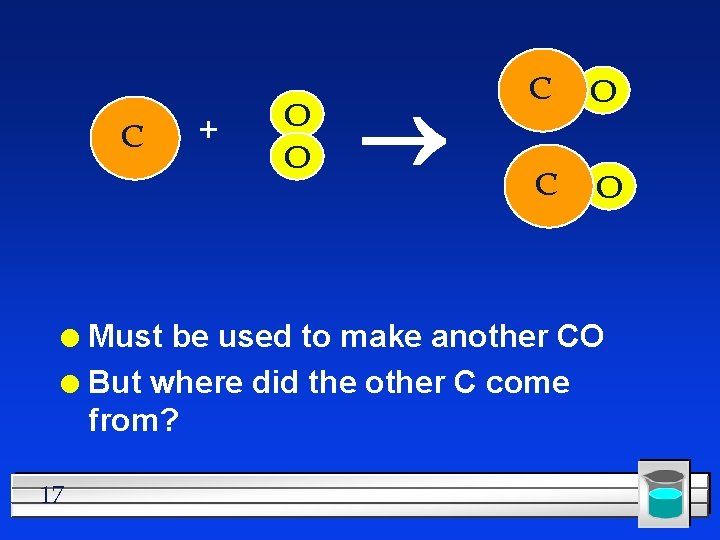C + O O C O Must be used to make another CO l But where did the other C come from? l 17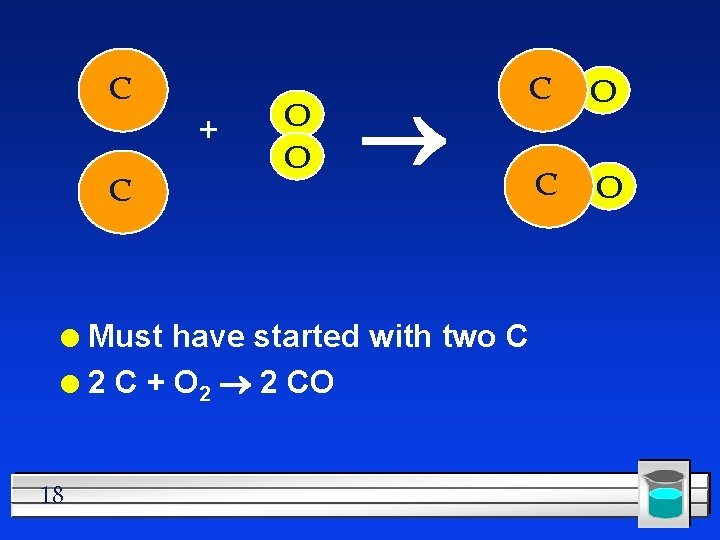C + C O O Must have started with two C l 2 C + O 2 2 CO l 18 C O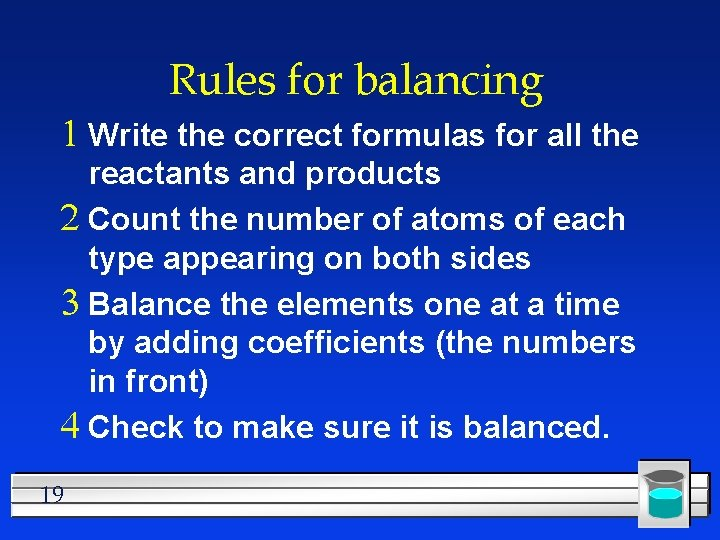Rules for balancing 1 Write the correct formulas for all the reactants and products 2 Count the number of atoms of each type appearing on both sides 3 Balance the elements one at a time by adding coefficients (the numbers in front) 4 Check to make sure it is balanced. 19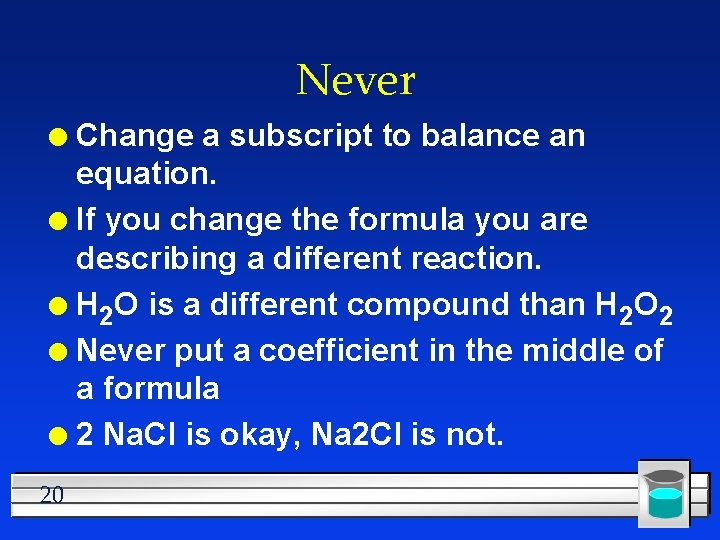Never Change a subscript to balance an equation. l If you change the formula you are describing a different reaction. l H 2 O is a different compound than H 2 O 2 l Never put a coefficient in the middle of a formula l 2 Na. Cl is okay, Na 2 Cl is not. l 20Example H 2 + O 2 H 2 O Make a table to keep track of where you are at 21Example H 2 + O 2 H 2 O R P 2 H 2 2 O 1 Need twice as much O in the product 22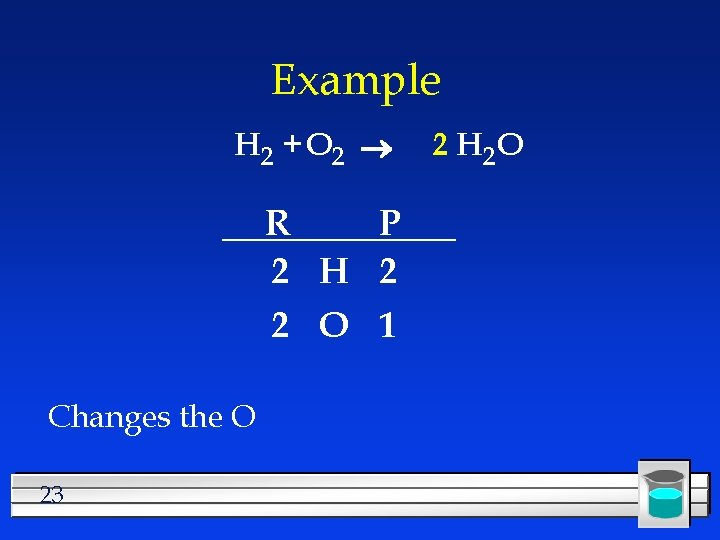Example H 2 + O 2 R P 2 H 2 2 O 1 Changes the O 23 2 H 2 O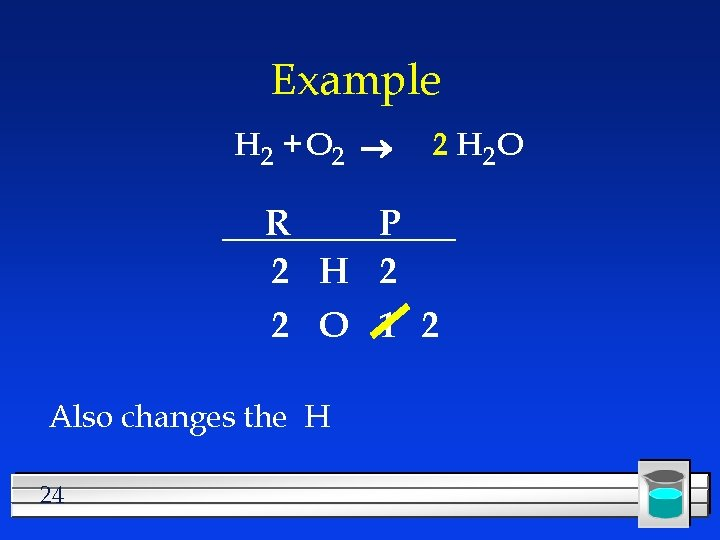Example H 2 + O 2 2 H 2 O R P 2 H 2 2 O 1 2 Also changes the H 24Example H 2 + O 2 2 H 2 O R P 2 H 2 4 2 O 1 2 Need twice as much H in the reactant 25Example 2 H 2 + O 2 2 H 2 O R P 2 H 2 4 2 O 1 2 Recount 26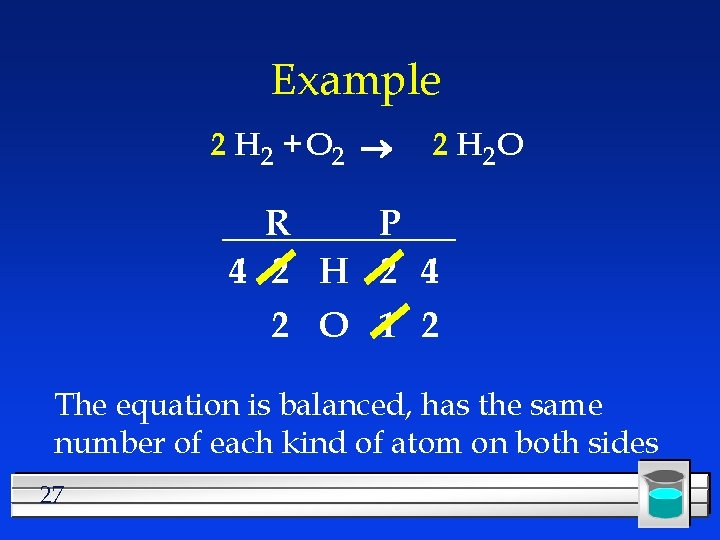Example 2 H 2 + O 2 2 H 2 O R P 4 2 H 2 4 2 O 1 2 The equation is balanced, has the same number of each kind of atom on both sides 27Example 2 H 2 + O 2 2 H 2 O R P 4 2 H 2 4 2 O 1 2 This is the answer Not this 28Examples CH 4 + O 2 CO 2 + H 2 O l Ag. NO 3 + Cu Cu(NO 3)2 + Ag l Mg + N 2 Mg 3 N 2 l P + O 2 P 4 O 10 l Na + H 2 O H 2 + Na. OH l 29Homework 14. a) Pb(NO 3)2 + K 2 Cr. O 4 Pb. Cr. O 4 + KNO 3 b) Mn. O 2 + HCl Mn. Cl 2 + H 2 O+ Cl 2 c) C 3 H 6 + O 2 CO 2 +H 2 O d) Zn(OH)2 + H 3 PO 4 Zn 3(PO 4)2 e) CO + Fe 2 O 3 Fe + CO 2 f) CS 2 + Cl 2 CCl 4 +S 2 Cl 2 g) CH 4 + Br 2 CH 3 Br + HBr h) Ba(CN)2 + H 2 SO 4 Ba. SO 4 + HCN 30Techniques If an atom appears more than once on a side, balance it last. l If you fix everything except one element, and it is even on one side and odd on the other, double the first number, then move on from there. l C 4 H 10 + O 2 CO 2 + H 2 O l 31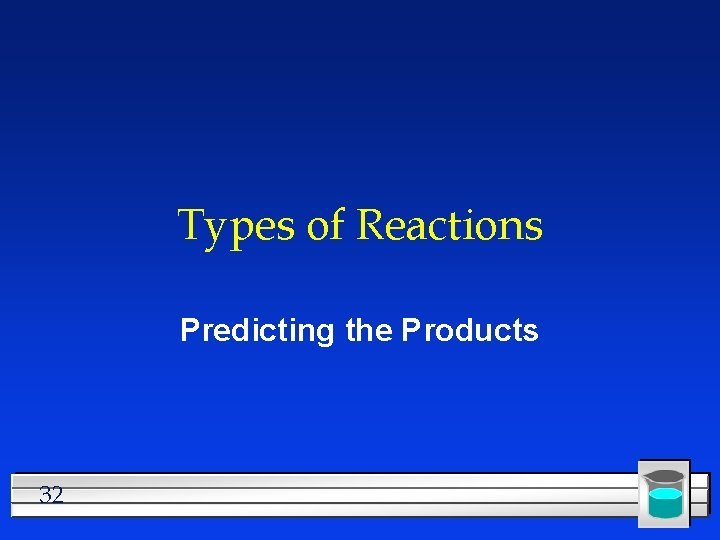Types of Reactions Predicting the Products 32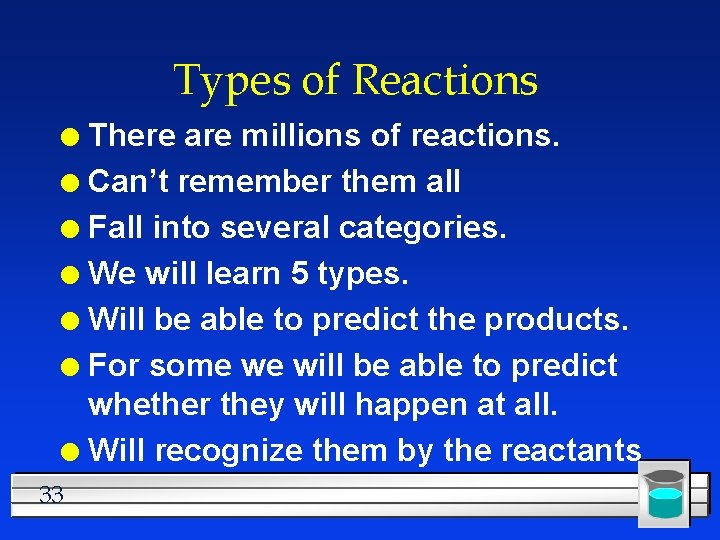Types of Reactions There are millions of reactions. l Can’t remember them all l Fall into several categories. l We will learn 5 types. l Will be able to predict the products. l For some we will be able to predict whether they will happen at all. l Will recognize them by the reactants l 33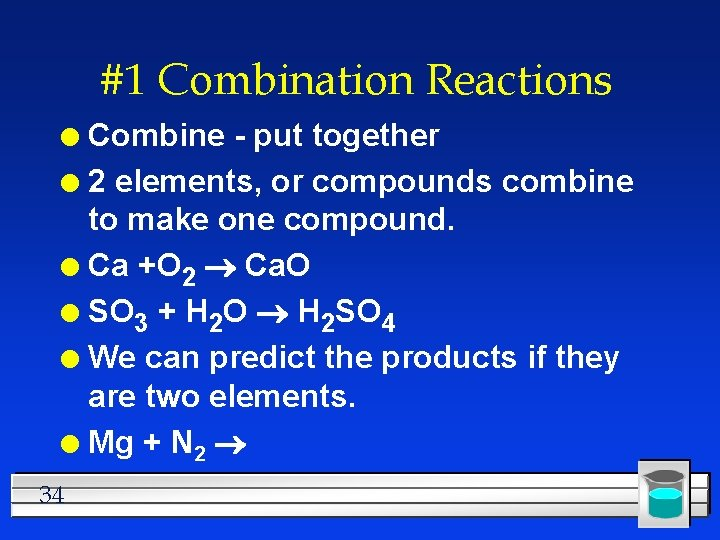#1 Combination Reactions Combine - put together l 2 elements, or compounds combine to make one compound. l Ca +O 2 Ca. O l SO 3 + H 2 O H 2 SO 4 l We can predict the products if they are two elements. l Mg + N 2 l 34Write and balance Ca + Cl 2 l Fe + O 2 iron (II) oxide l Al + O 2 l Remember that the first step is to write the formula l Then balance l 35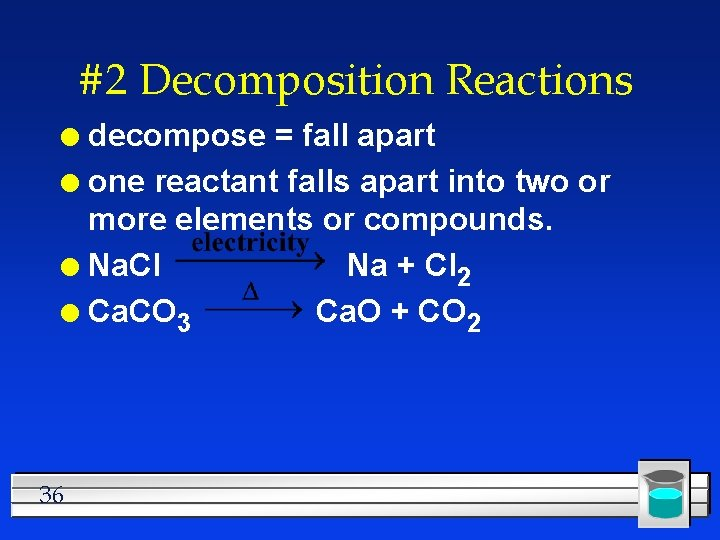#2 Decomposition Reactions decompose = fall apart l one reactant falls apart into two or more elements or compounds. l Na. Cl Na + Cl 2 l Ca. CO 3 Ca. O + CO 2 l 36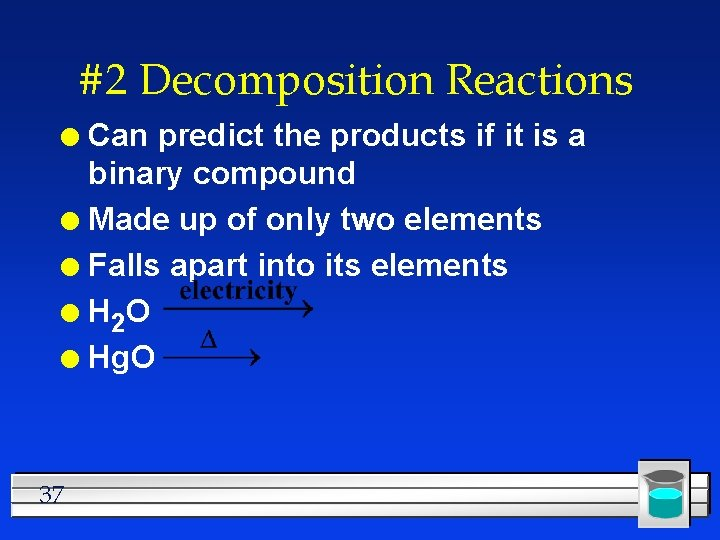#2 Decomposition Reactions Can predict the products if it is a binary compound l Made up of only two elements l Falls apart into its elements l H 2 O l Hg. O l 37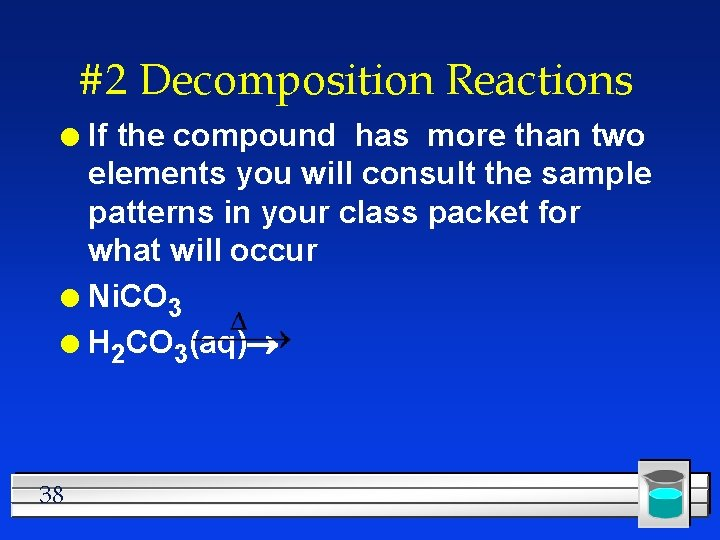#2 Decomposition Reactions If the compound has more than two elements you will consult the sample patterns in your class packet for what will occur l Ni. CO 3 l H 2 CO 3(aq) l 38#3 Single Replacement One element replaces another l Reactants must be an element and a compound. l Products will be a different element and a different compound. l Na + KCl K + Na. Cl l F 2 + Li. Cl Li. F + Cl 2 l 39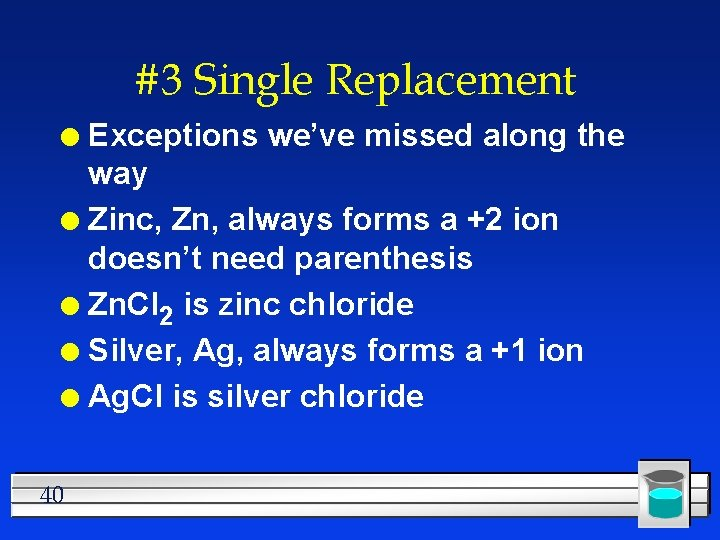#3 Single Replacement Exceptions we’ve missed along the way l Zinc, Zn, always forms a +2 ion doesn’t need parenthesis l Zn. Cl 2 is zinc chloride l Silver, Ag, always forms a +1 ion l Ag. Cl is silver chloride l 40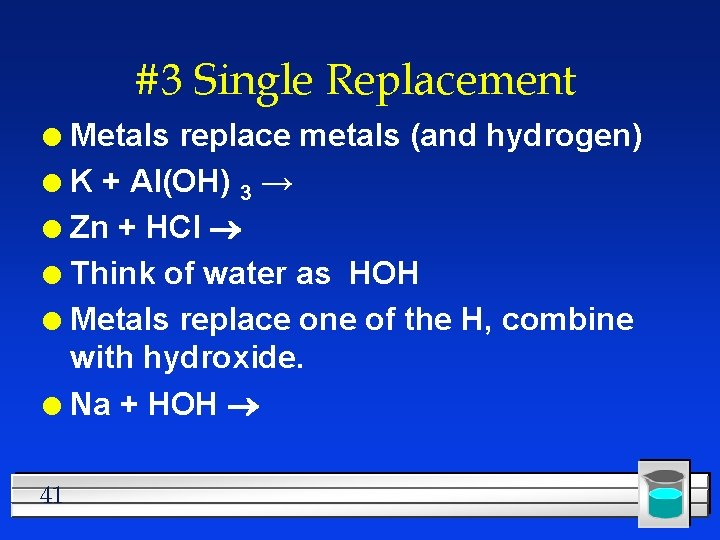#3 Single Replacement Metals replace metals (and hydrogen) l K + Al(OH) 3 → l Zn + HCl l Think of water as HOH l Metals replace one of the H, combine with hydroxide. l Na + HOH l 41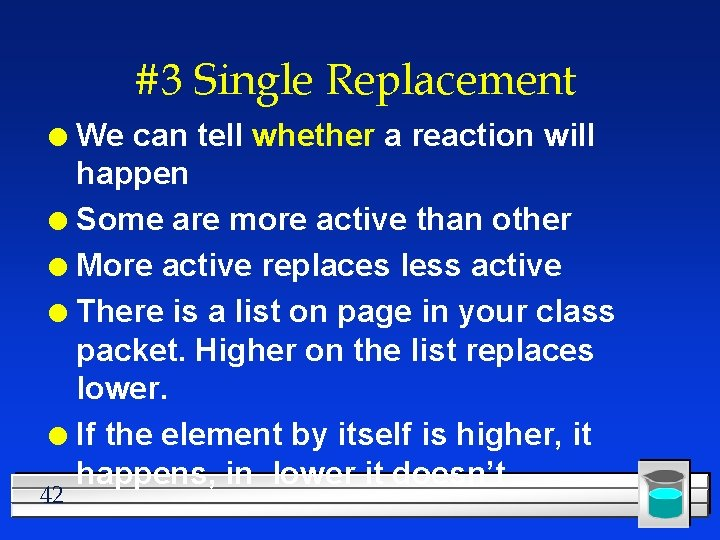#3 Single Replacement We can tell whether a reaction will happen l Some are more active than other l More active replaces less active l There is a list on page in your class packet. Higher on the list replaces lower. l If the element by itself is higher, it happens, in lower it doesn’t l 42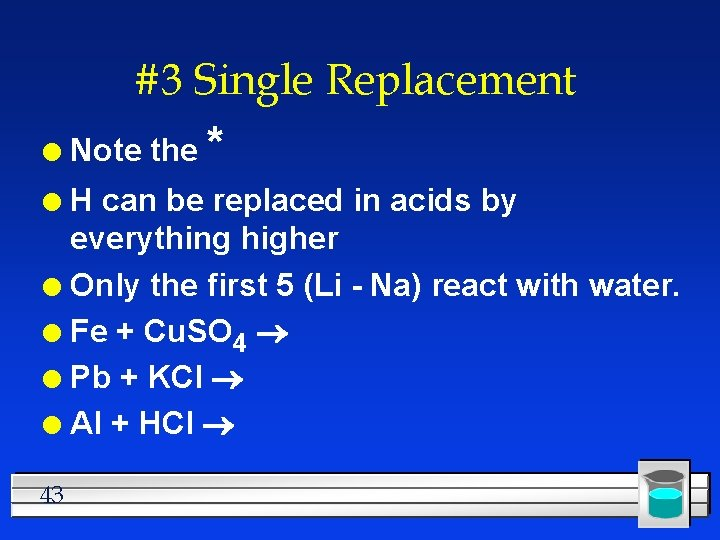#3 Single Replacement l Note the * H can be replaced in acids by everything higher l Only the first 5 (Li - Na) react with water. l Fe + Cu. SO 4 l Pb + KCl l Al + HCl l 43#3 Single Replacement What does it mean that Au and Ag are on the bottom of the list? l Nonmetals can replace other nonmetals l Limited to F 2 , Cl 2 , Br 2 , I 2 l The order of activity is that on the table. l Higher replaces lower. l F 2 + HCl l Br 2 + KCl l 44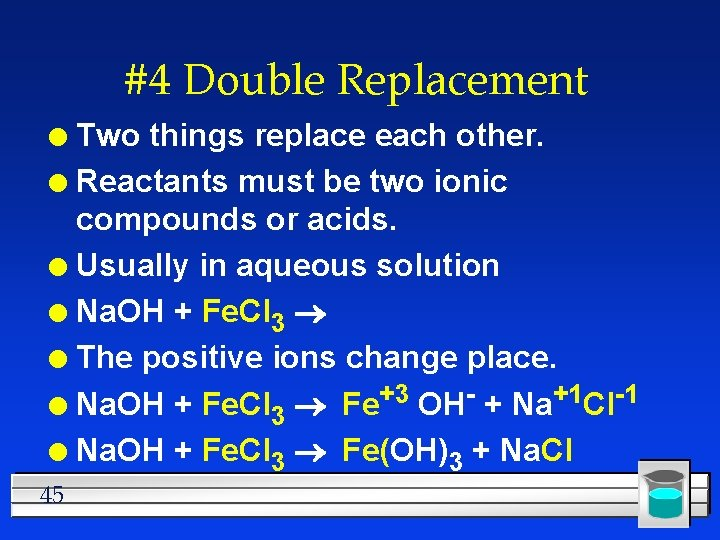#4 Double Replacement Two things replace each other. l Reactants must be two ionic compounds or acids. l Usually in aqueous solution l Na. OH + Fe. Cl 3 l The positive ions change place. l Na. OH + Fe. Cl 3 Fe+3 OH- + Na+1 Cl-1 l Na. OH + Fe. Cl 3 Fe(OH)3 + Na. Cl l 45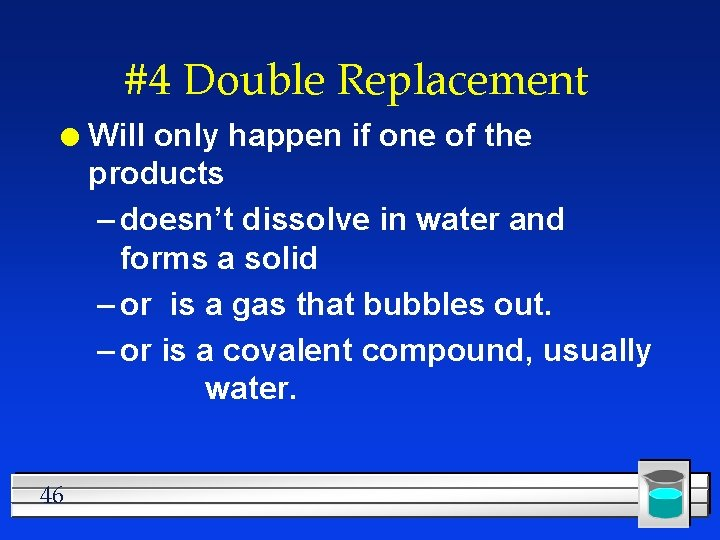#4 Double Replacement l 46 Will only happen if one of the products – doesn’t dissolve in water and forms a solid – or is a gas that bubbles out. – or is a covalent compound, usually water.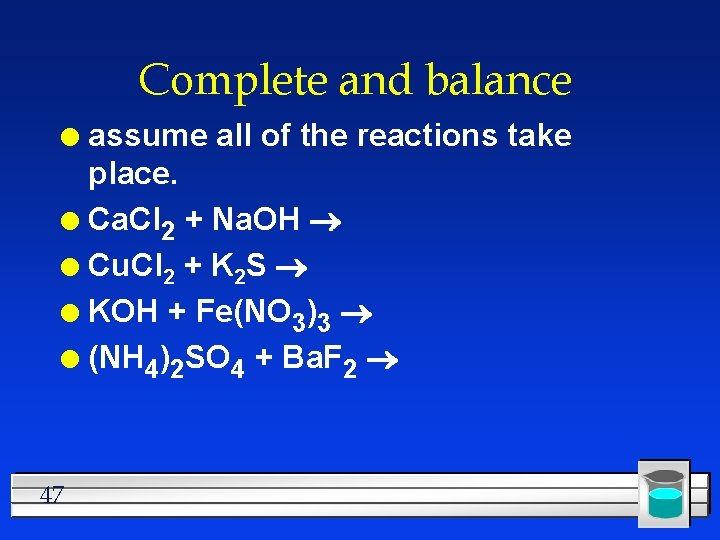Complete and balance assume all of the reactions take place. l Ca. Cl 2 + Na. OH l Cu. Cl 2 + K 2 S l KOH + Fe(NO 3)3 l (NH 4)2 SO 4 + Ba. F 2 l 47How to recognize which type Look at the reactants l. E+E Combination l. C Decomposition l E + C Single replacement l C + C Double replacement l 48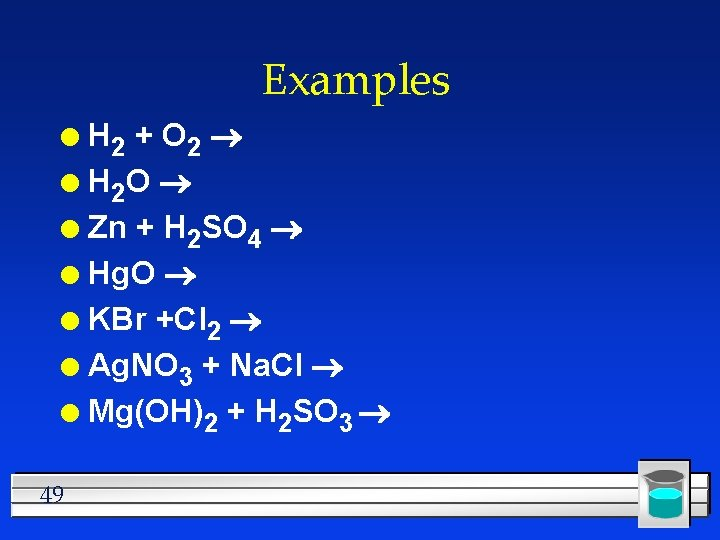Examples H 2 + O 2 l H 2 O l Zn + H 2 SO 4 l Hg. O l KBr +Cl 2 l Ag. NO 3 + Na. Cl l Mg(OH)2 + H 2 SO 3 l 49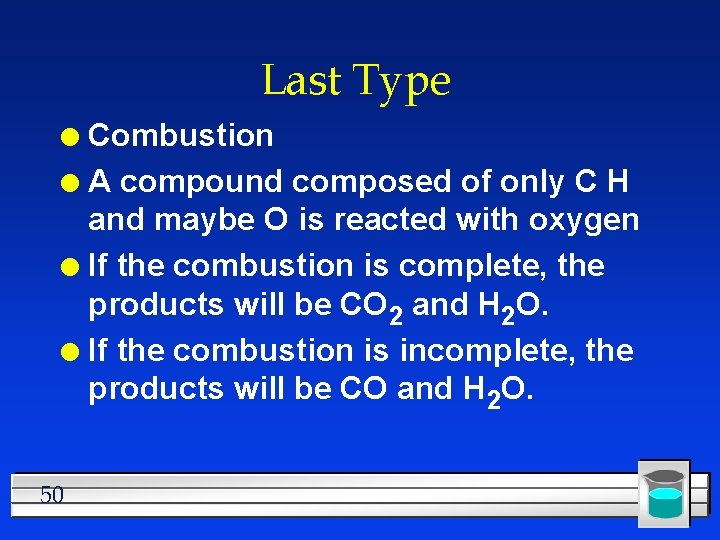Last Type Combustion l A compound composed of only C H and maybe O is reacted with oxygen l If the combustion is complete, the products will be CO 2 and H 2 O. l If the combustion is incomplete, the products will be CO and H 2 O. l 50Examples C 4 H 10 + O 2 (complete) l C 4 H 10 + O 2 (incomplete) l C 6 H 12 O 6 + O 2 (complete) l C 8 H 8 +O 2 (incomplete) l 51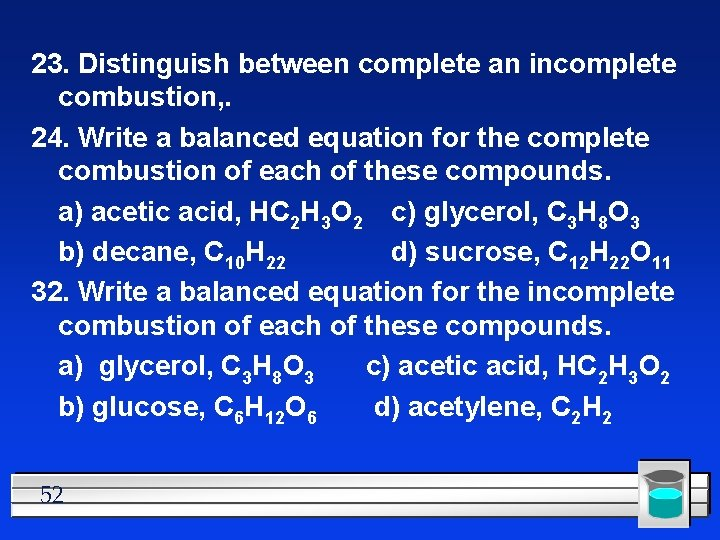23. Distinguish between complete an incomplete combustion, . 24. Write a balanced equation for the complete combustion of each of these compounds. a) acetic acid, HC 2 H 3 O 2 c) glycerol, C 3 H 8 O 3 b) decane, C 10 H 22 d) sucrose, C 12 H 22 O 11 32. Write a balanced equation for the incomplete combustion of each of these compounds. a) glycerol, C 3 H 8 O 3 c) acetic acid, HC 2 H 3 O 2 b) glucose, C 6 H 12 O 6 d) acetylene, C 2 H 2 52Chapter 7 Summary 53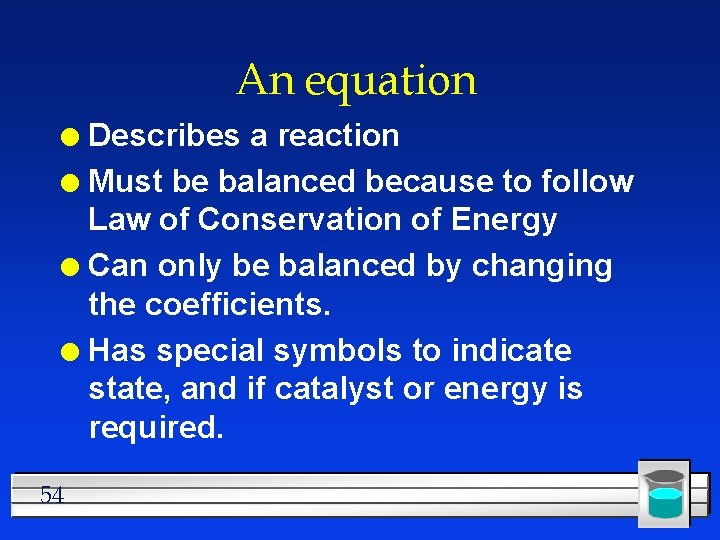An equation Describes a reaction l Must be balanced because to follow Law of Conservation of Energy l Can only be balanced by changing the coefficients. l Has special symbols to indicate state, and if catalyst or energy is required. l 54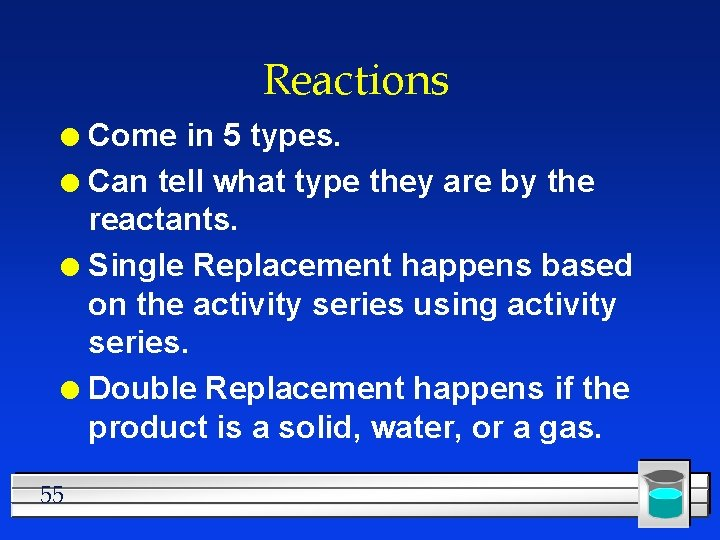Reactions Come in 5 types. l Can tell what type they are by the reactants. l Single Replacement happens based on the activity series using activity series. l Double Replacement happens if the product is a solid, water, or a gas. l 55The Process Determine the type by looking at the reactants. l Put the pieces next to each other l Use charges to write the formulas l Use coefficients to balance the equation. l 56 Do your Homework